\$B:#>,\$N0lGU(B

\$BKhF|!":#>,\$N0lGU\$r>e\$2\$F\$\$\$^\$9!#(B
\$B:#>,0{\$`\$b\$N\$K:\$\$C\$?\$i!";29M\$K\$7\$F\$_\$F\$/\$@\$5\$\$!#(B

2020\$BG/(B9\$B7n(B

 1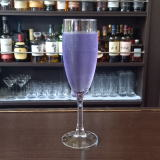(I1R<^=D(B 2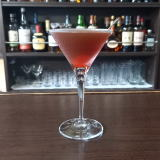(I;]LW]<=:(B 3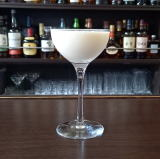(I=20D%OX1(B 4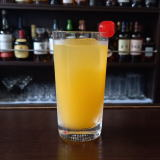(I<^*]DY%M^](B 5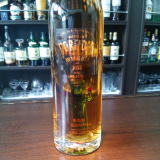(IN_YL(C^(5\$B!!(I1HN(B 6 \$B5Y\$_(B 7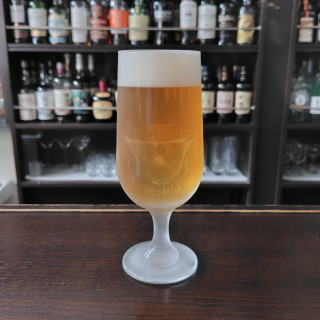(IN^2W0%R060(B 8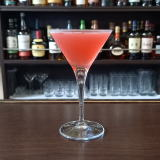(IZC^(%W28(B 9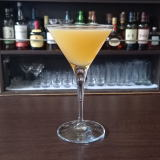(IZ/D%J^DW0(B 10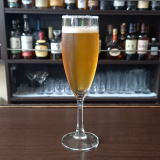(I<6:^(B 11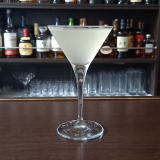(I 12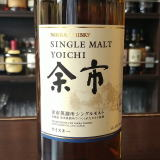\$BM>;T(B 13 \$B5Y\$_(B 14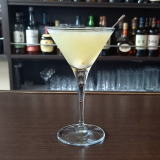(I<^-4Y%J3=(B 15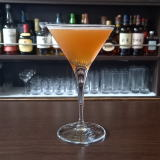(I\<]D](B 16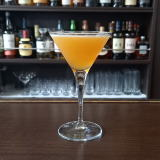(I<^.X0%[<^,0(B 17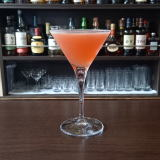(I=60Z/D%5JW(B 18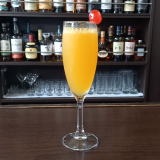(I@^2EO2D(B 19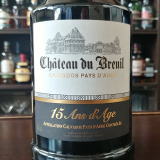(I<,D0%D^%L^Y2U(B15\$BG/(B 20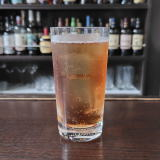(I8^X0]1/L_Y<^]<^,0(B 21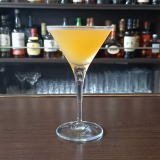(I90%50%68CY(B 22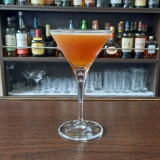(I@^0C(0%JX0(B 23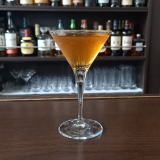(I50%M]X0(B 24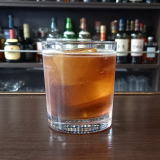(IL^W/8%35/A(B 25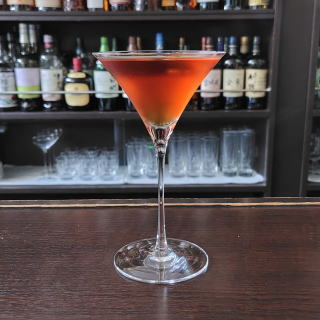(I[0Y=%[2=(B 26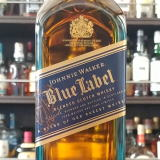(I<^.F03+060\$B!&(IL^Y0(B 27 \$B5Y\$_(B 28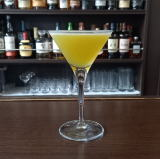(I50YD^%7,/=Y(B 29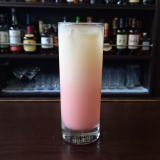(INLO]%L(=^(B 30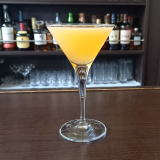(I:0WY(B